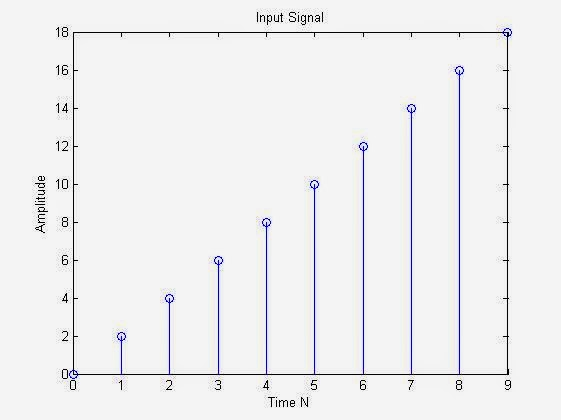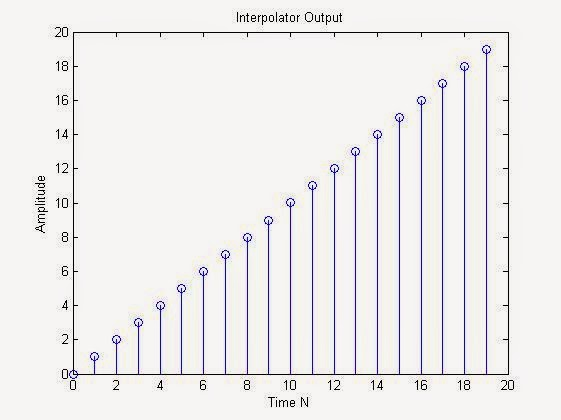# Upsampling & Interpolation of Discrete Signal/Sequence using MATLAB : MATLAB code for Upsampling & Interpolation

Upsampling is a process of inserting zero-amplitude samples between original samples to increase the sampling rate. (also called zero stuffing) whereas Interpolation is the process of upsampling followed by filtering(Low Pass) to create in between samples from the original spaced samples.It can be just viewed that we are adding the average value of the original sample in place of zero valued samples.Up-sampling & Interpolating of Original Signal using MATLAB : MATLAB code for Upsampling & Interpolation
MATLAB code for Up-sampling & Interpolation
clc;
close all;
clear all;
n=input('Enter length of input sequence:');
l=input('Enter up-sampling factor:');

m=0:n-1; %making a vector m from the lenght of input sequence
a=input('Enter Slope of Ramp Signal:');
x = (0:n-1)*a;
% for generating ramp signal x with a slope of a
%replace your own signal/sequence with the variable x

figure,stem(m,x);
xlabel('Time N');
ylabel('Amplitude');
title('Input Signal');

lx = n;
y = 0;
y(1) = x(1);
for i=2:lx
c = [zeros(1,l-1),x(i)];
%inserting zeros in between original samples
y = [y,c];
end

nm=0:length(y)-1;
figure,stem(nm,y);
%Upsampled version of signal
xlabel('Time N');
ylabel('Amplitude');
title('Up-Sampled Output of Signal');

xi=x;

yi = interp(xi,l);
nn=0:length(yi)-1;
figure,stem(nn,yi);
%interpolation output
xlabel('Time N');
ylabel('Amplitude');
title('Interpolator Output');

Sample Input through MATLAB command Window:

Enter length of input sequence:10
Enter up-sampling factor:2
Enter Slope of Ramp Signal:2

Output of above Inputs:Original Input Signal : MATLAB PlotUp-sampled Version of the signal : MATLAB PlotUp-sampled & Interpolated Version of the signal : MATLAB Plot

Find more at Digital iVision Labs!

# Implementation of RSA Cryptography Algorithm Using MATLAB Code
# Recording a Video From Webcam Using MATLAB Code

# Accessing the Serial Port using MATLAB Code: Serial communication through MATLAB
# Accessing Webcam Through MATLAB Code
# Motion Tracking/Detection in MATLAB using Kalman Filter
# Optimizing MATLAB Code
# Controlling Mouse Pointer/Cursor Using MATLAB Code
# Controlling Keyboard Keys (Pressing & Releasing) with Matlab code
# Starting MATLAB without GUI through Command Prompt/LxTERMINAL
# Some MATLAB Unusual Commands, That You Must Know ( Just For Fun! ) Part 2
# Some MATLAB Unusual Commands, That You Must Know ( Just For Fun! ) Part 1